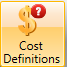FIN

Cost Definition Dialog
 What Cost definitions setup Where EarthCost formula Dialog
 What Formula setup for cost calculation Where EarthCost Wizard Dialog
 What Cost calculation wizard Where EarthExchange Rates Dialog
 What Exchange rates used for cos calculation Where EarthDid you find it helpful? Yes No

Send feedback